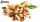Roses

On the large rosary was a third white, half red, yellow quarter and six pink. How many roses was in the rosary?

Result

x =  -72

Solution:

x/3+x/2+x/4+6=x

x = -72

x = -72

Calculated by our simple equation calculator.

Leave us a comment of this math problem and its solution (i.e. if it is still somewhat unclear...):Be the first to comment!To solve this verbal math problem are needed these knowledge from mathematics:

Need help calculate sum, simplify or multiply fractions? Try our fraction calculator. Do you have a linear equation or system of equations and looking for its solution? Or do you have quadratic equation?

Next similar math problems:

1. One thirdIf 3/5 is 360, how much is 1/3?
2. Unknown numberI think the number - its sixth is 3 smaller than its third.
3. Sales storesThe first sales store passed the 1/3 and the second 2/5 of the total amount of goods.In third store passed the 2/3 rest of the goods. The remaining 40 kg of goods put into fourth store. How many kilograms of goods pass to a third store?
4. UN 1If we add to an unknown number his quarter, we get 210. Identify unknown number.
5. FractionsThree-quarters of an unknown number are 4/5. What is 5/6 of this unknown number?
6. CandyPeter had a sachet of candy. He wanted to share with his friends. If he gave them 30 candies, he would have 62 candies. If he gave them 40 candies, he would miss 8 candies. How many friends did Peter have?
7. PearsThere were pears in the basket, I took two-fifths of them, and left six in the basket. How many pears did I take?
8. Equation with fractionsSolve equation: ? It is equation with fractions.
9. EqnSolve equation with fractions: 2x/3-50=40+x/4
10. Fraction + eqSolve following simple equation with fractions: -5/6(8+5b) = 75 + 5/3b
11. Eq-fracSolve the following equation with fractions: h + 1/3 =5/3
12. Equation with mixed fractions2 3/5 of 1430+? = 1900. How to do this problem
13. Simple equationSolve for x: 3(x + 2) = x - 18
14. Fifth of the numberThe fifth of the number is by 24 less than that number. What is the number?
15. Equation with xSolve the following equation: 2x- (8x + 1) - (x + 2) / 5 = 9
16. Bag of peanutsJoe eat 1/3 of a bag of peanuts, mark eat 1/4 of the remaining in the bag of peanuts, Alvin eat 1/2 of the remaining bag of peanuts, peter eat 10 peanuts, there are 71 peanuts left. Hon many peanuts were in the bags?
17. EquationSolve the equation: 1/2-2/8 = 1/10; Write the result as a decimal number.